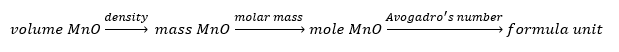# Problem: MnO has either the NaCl type structure or the CsCl type structure. The edge length of the MnO unit cell is 4.47 x 10-8 cm and the density of MnO is 5.28 g/cm 3. Assuming that the ionic radius of oxygen is 140. pm, estimate the ionic radius of manganese.

###### FREE Expert Solution

We need to identify the type of structure of MnO by determining how many atoms are present on the MnO unit cell.Given:

Edge length (a) = 4.47x10-8 cm
Density = 5.28 g/cm3

Let’s first calculate the volume of the unit cell:

88% (119 ratings)###### Problem Details

MnO has either the NaCl type structure or the CsCl type structure. The edge length of the MnO unit cell is 4.47 x 10-8 cm and the density of MnO is 5.28 g/cm 3. Assuming that the ionic radius of oxygen is 140. pm, estimate the ionic radius of manganese.